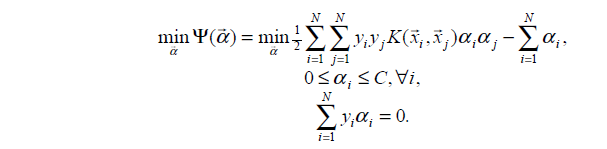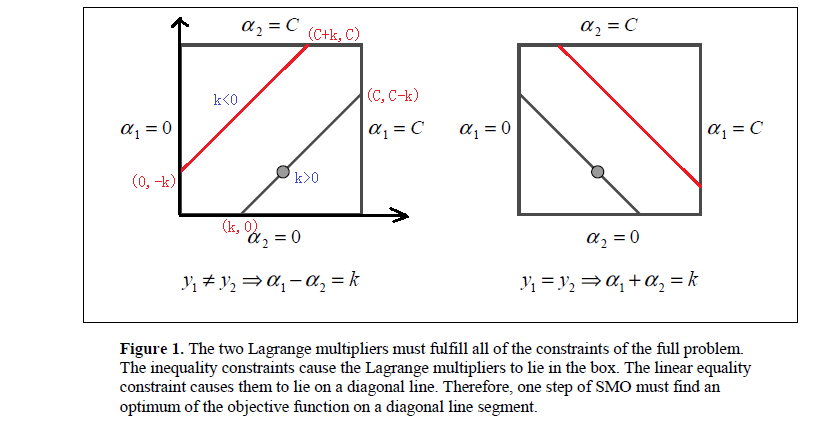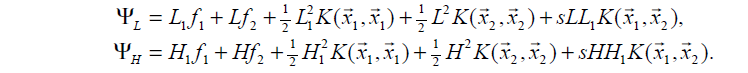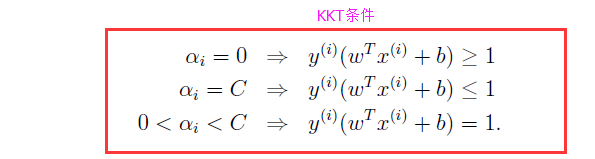# 【机器学习详解】SMO算法剖析Machine Learning 同时被 2 个专栏收录10 篇文章 3 订阅
CSDN

# 1.SMO概念# 2.SMO原理分析

## 2.1视为一个二元函数

SMO算法选择同时优化两个参数，固定其他N-2个参数，假设选择的变量为 α1,α2 $\alpha_1,\alpha_2$,固定其他参数 α3,α4,...,αN $\alpha_3,\alpha_4,...,\alpha_N$,由于参数 α3,α4,...,αN $\alpha_3,\alpha_4,...,\alpha_N$的固定，可以简化目标函数为只关于 α1,α2 $\alpha_1,\alpha_2$的二元函数， Constant $Constant$表示常数项(不包含变量 α1,α2 $\alpha_1,\alpha_2$的项)。

min Ψ(α1,α2)=12K11α21+12K22α22+y1y2K12α1α2(α1+α2)+y1v1α1+y2v2α2+Constant(1)

## 2.2视为一元函数

α1=(ζy2α2)y1(2)
(2)式带回到(1)中得到只关于参数 α2 $\alpha_2$的一元函数，由于常数项不影响目标函数的解，以下省略掉常数项 Constant $Constant$
min Ψ(α2)=12K11(ζα2y2)2+12K22α22+y2K12(ζα2y2)α2(ζα2y2)y1α2+v1(ζα2y2)+y2v2α2(3)

## 2.3对一元函数求极值点

Ψ(α2)α2=(K11+K222K12)α2K11ζy2+K12ζy2+y1y21v1y2+v2y2=0 $\frac{\partial \Psi (\alpha_2)}{\partial \alpha_2}=(K_{11}+K_{22}-2K_{12})\alpha_2-K_{11}\zeta y_2+K_{12}\zeta y_2+y_1y_2-1-v_1y_2+v_2y_2=0$

1.由上式中假设求得了 α2 $\alpha_2$的解，带回到(2)式中可求得 α1 $\alpha_1$的解，分别记为 αnew1,αnew2 $\alpha_1^{new},\alpha_2^{new}$,优化前的解记为 αold1,αold2 $\alpha_1^{old},\alpha_2^{old}$;由于参数 α3,α4,...,αN $\alpha_3,\alpha_4,...,\alpha_N$固定，由等式约束 Ni=1yiαi=0 $\sum_{i=1}^{N}y_i\alpha_i=0$ αold1y1+αold2y2=Ni=3αiyi=αnew1y1+αnew2y2=ζ $\alpha_1^{old}y_1+\alpha_2^{old}y_2=-\sum_{i=3}^N\alpha_iy_i=\alpha_1^{new}y_1+\alpha_2^{new}y_2=\zeta$

ζ=αold1y1+αold2y2(4)

2.假设SVM超平面的模型为 f(x)=wTx+b $f(x)=w^Tx+b$,上一篇中已推导出 w $w$的表达式,将其带入得f(x)=Ni=1αiyiK(xi,x)+b;f(xi)$f(x)=\sum_{i=1}^N\alpha_iy_iK(x_i,x)+b;f(x_i)$表示样本 xi $x_i$的预测值, yi $y_i$表示样本 xi $x_i$的真实值，定义 Ei $E_i$表示预测值与真实值之差为
Ei=f(xi)yi(5)

3.由于 vi=Nj=3αjyjK(xi,xj),i=1,2 $v_i=\sum_{j=3}^N\alpha_jy_jK(x_i,x_j),i=1,2$，因此
v1=f(x1)j=12yjαjK1jb(6)

v2=f(x2)j=12yjαjK2jb(7)

(K11+K222K12)α2K11ζy2+K12ζy2+y1y21v1y2+v2y2=0 $(K_{11}+K_{22}-2K_{12})\alpha_2-K_{11}\zeta y_2+K_{12}\zeta y_2+y_1y_2-1-v_1y_2+v_2y_2=0$

(K11+K222K12)αnew,unclipped2=(K11+K222K12)αold2+y2[y2y1+f(x1)f(x2)] $(K_{11}+K_{22}-2K_{12})\alpha_2^{new,unclipped}=(K_{11}+K_{22}-2K_{12})\alpha_2^{old}+y_2\left[y_2-y_1+f(x_1)-f(x_2)\right]$

αnew,unclipped2=αold2+y2(E1E2)η(8)

## 2.4对原始解修剪

• 0αi=1,2C $0\leq\alpha_{i=1,2}\leq C$
• α1y1+α2y2=ζ $\alpha_1y_1+\alpha_2y_2=\zeta$y1y2 $y_1\neq y_2$时， L=max(0,αold2αold1);H=min(C,C+αold2αold1) $L=max(0,\alpha_2^{old}-\alpha_1^{old});H=min(C,C+\alpha_2^{old}-\alpha_1^{old})$
y1=y2 $y_1= y_2$时， L=max(0,αold1+αold2C);H=min(C,αold2+αold1) $L=max(0,\alpha_1^{old}+\alpha_2^{old}-C);H=min(C,\alpha_2^{old}+\alpha_1^{old})$

αnew2= H ,αnew,unclipped2>Hαnew,unclipped2,Lαnew,unclipped2H L ,αnew,unclipped2<L

## 2.5求解 αnew1 $\alpha_1^{new}$

αnew1=αold1+y1y2(αold2αnew2)(9)

## 2.6取临界情况

1. η<0 $\eta<0$,当核函数K不满足Mercer定理时，矩阵K非正定；
2. η=0 $\eta=0$,样本 x1 $x_1$ x2 $x_2$输入特征相同；

η<0 $\eta<0$时，目标函数为凸函数，没有极小值，极值在定义域边界处取得。
η=0 $\eta=0$时，目标函数为单调函数，同样在边界处取极值。# 3.启发式选择变量

## 第一个变量的选择## 第二个变量的选择

SMO称第二个变量的选择过程为内循环，假设在外循环中找个第一个变量记为 α1 $\alpha_1$，第二个变量的选择希望能使 α2 $\alpha_2$有较大的变化，由于 α2 $\alpha_2$是依赖于 |E1E2| $|E_1-E_2|$,当 E1 $E_1$为正时，那么选择最小的 Ei $E_i$作为 E2 $E_2$,如果 E1 $E_1$为负，选择最大 Ei $E_i$作为 E2 $E_2$，通常为每个样本的 Ei $E_i$保存在一个列表中，选择最大的 |E1E2| $|E_1-E_2|$来近似最大化步长。

# 4.阈值b的计算

1.如果 0<αnew1<C $0<\alpha_1^{new}，由KKT条件 y1(wTx1+b)=1 $y_1(w^Tx_1+b)=1$,得到 Ni=1αiyiKi1+b=y1 $\sum_{i=1}^N\alpha_iy_iK_{i1}+b=y_1$,由此得：

bnew1=y1i=3NαiyiKi1αnew1y1K11αnew2y2K21

y1i=3NαiyiKi1=E1+αold1y1K11+αold2y2K11+bold

bnew1=E1y1K11(αnew1αold1)y2K21(αnew2αold2)+bold

2.如果 0<αnew2<C $0<\alpha_2^{new}，则
bnew2=E2y1K12(αnew1αold1)y2K22(αnew2αold2)+bold

3.如果同时满足 0<αnewi<C $0<\alpha_i^{new},则 bnew1=bnew2 $b_1^{new}=b_2^{new}$
4.如果同时不满足 0<αnewi<C $0<\alpha_i^{new}，则 bnew1bnew2 $b_1^{new}与b_2^{new}$以及它们之间的数都满足KKT阈值条件，这时选择它们的中点。（ 关于这个我不理解…

Sequential Minimal Optimization:A Fast Algorithm for Training Support Vector Machines，John C. Platt

11-13833607-1415
07-231万+
05-30277
06-185万+
06-17608
10-192553
01-24110
02-271169
06-30
01-26515
07-19258
08-151689
06-26141
09-22195
08-173万+
10-24210点击重新获取扫码支付余额充值